# CLASS 10 MATH NCERT SOLUTIONS FOR CHAPTER – 13 Surface Areas and Volumes Ex – 13.1

## Surface Areas and Volumes

Question 1.
2 cubes each of volume 64 cm3 are joined end to end. Find the surface area of the resulting cuboid.

Solution:
Let the length of side of the cube be a cm.
Then, its volume = a3 cm3   ⇒  a3 = 64   [Given]
⇒ a =$\sqrt [ 3 ]{ 64 }$ = 4 cm
By joining the two cubes, a cuboid is formed withe length, breadth and height 8 cm, 4 cm and 4 cm respectively.
∴ Surface area of the cuboid = 9(lb + bh + hl)
= 2(8 x 4 + 4 x 4 + 4 x 8) cm2
= 2(32 + 16 + 32) = 160 cm

Question 2.
A vessel is in the form of a hollow hemisphere mounted by a hollow cylinder. The diameter of the hemisphere is 14 cm and the total height of the vessel is 13 cm. Find the inner surface area of the vessel.

Solution:
Radius of hemisphere, r =$\frac { 14 }{ 2 }$ = 7 cm
Height of cylinder, h = 13 – 7 = 6 cm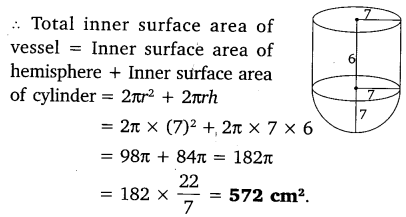Question 3.
A toy is in the form of a cone of radius 3.5 cm mounted on a hemisphere of same radius. The total height of the toy is 15.5 cm. Find the total surface area of the toy.

Solution:
Radius of cone, r = 3.5 cm
Height of cone, h = 15.5 – 3.5 = 12 cm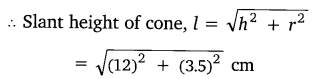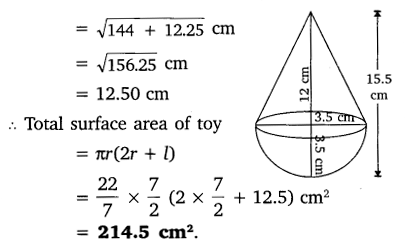Question 4.
A cubical block of side 7 cm is surmounted by a hemisphere. What is the greatest diameter the hemisphere can have? Find the surface area of the solid.

Solution: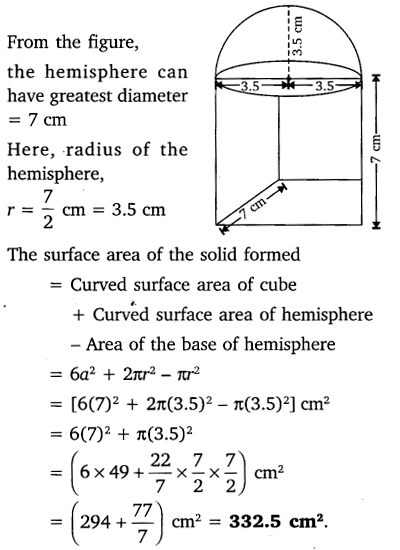Question 5.
A hemispherical depression is cut out from one face of a cubical wooden block such that the diameter l of the hemisphere is equal to the edge of the cube. Determine the surface area of the remaining solid.

Solution:
Diameter of hemisphere = edge of cube = l
So the radius of hemisphere =$\frac { l }{ 2 }$Question 6.
A medicine capsule is in the shape of a cylinder with two hemispheres stuck to each of its ends (see figure). The length of the entire capsule is 14 mm and the diameter of the capsule is 5 mm. Find its surface area.

Solution:
Radius of cylinder, r = 2.5 mm
Length of cylinder, h = 9 mmQuestion 7.
A tent is in the shape of a cylinder surmounted by a conical top. If the height and diameter of the cylindrical part are 2.1 m and 4 m respectively, and the slant height of the top is 2.8 m, find the area of the canvas used for making the tent. Also, find the cost of the canvas of the tent at the rate of ₹ 500 per m². (Note that the base of the tent will not be covered with canvas.)

Solution: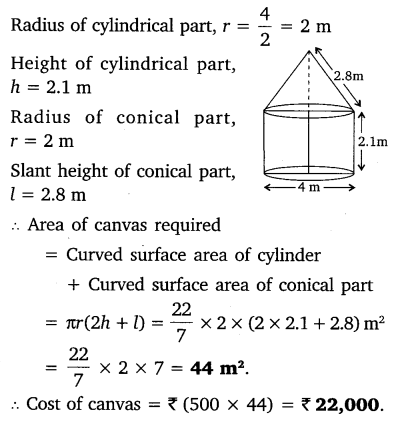Question 8.
From a solid cylinder whose height is 2.4 cm and diameter 1.4 cm, a conical cavity of the same height and same diameter is hollowed out. Find the total surface area of the remaining solid to the nearest cm².

Solution:Question 9.
A wooden article was made by scooping out a hemisphere from each end of a solid cylinder, as shown in figure. If the height of the cylinder is 10 cm, and its base is of radius 3.5 cm, find the total surface area of the article.

Solution:#### CLASS 10 MATH NCERT SOLUTIONS FOR CHAPTER – 13 SURFACE AREAS AND VOLUMES EX – 13.2

Lorem ipsum dolor sit amet, consectetur adipiscing elit. Phasellus cursus rutrum est nec suscipit. Ut et ultrices nisi. Vivamus id nisl ligula. Nulla sed iaculis ipsum.

Company Name